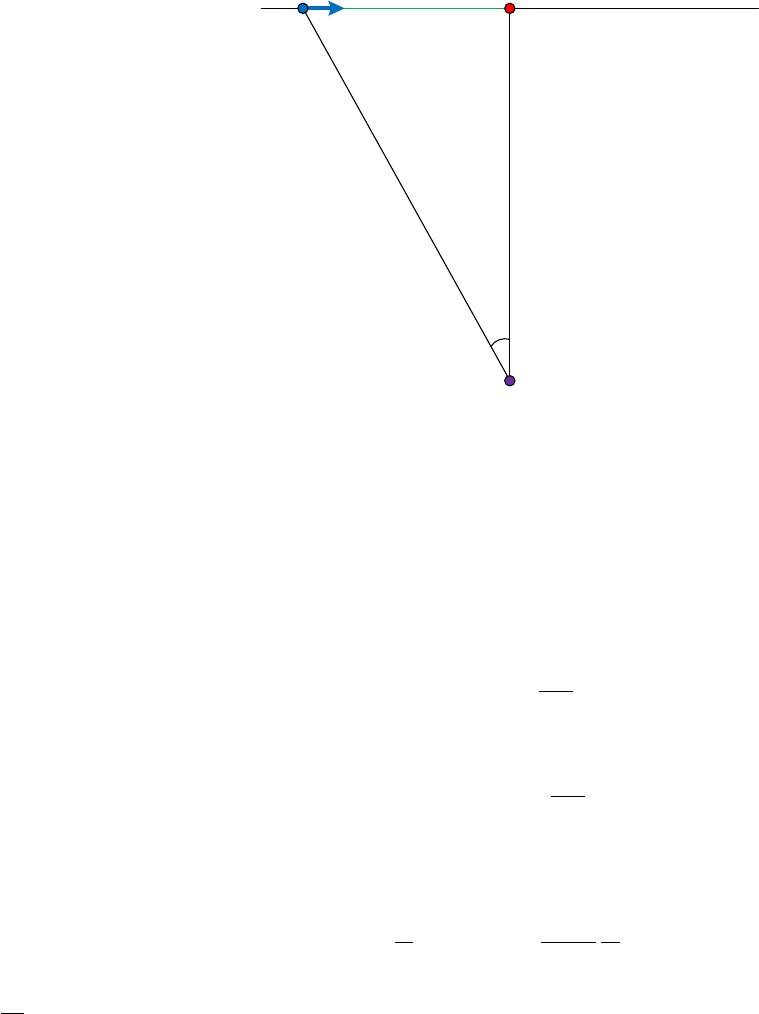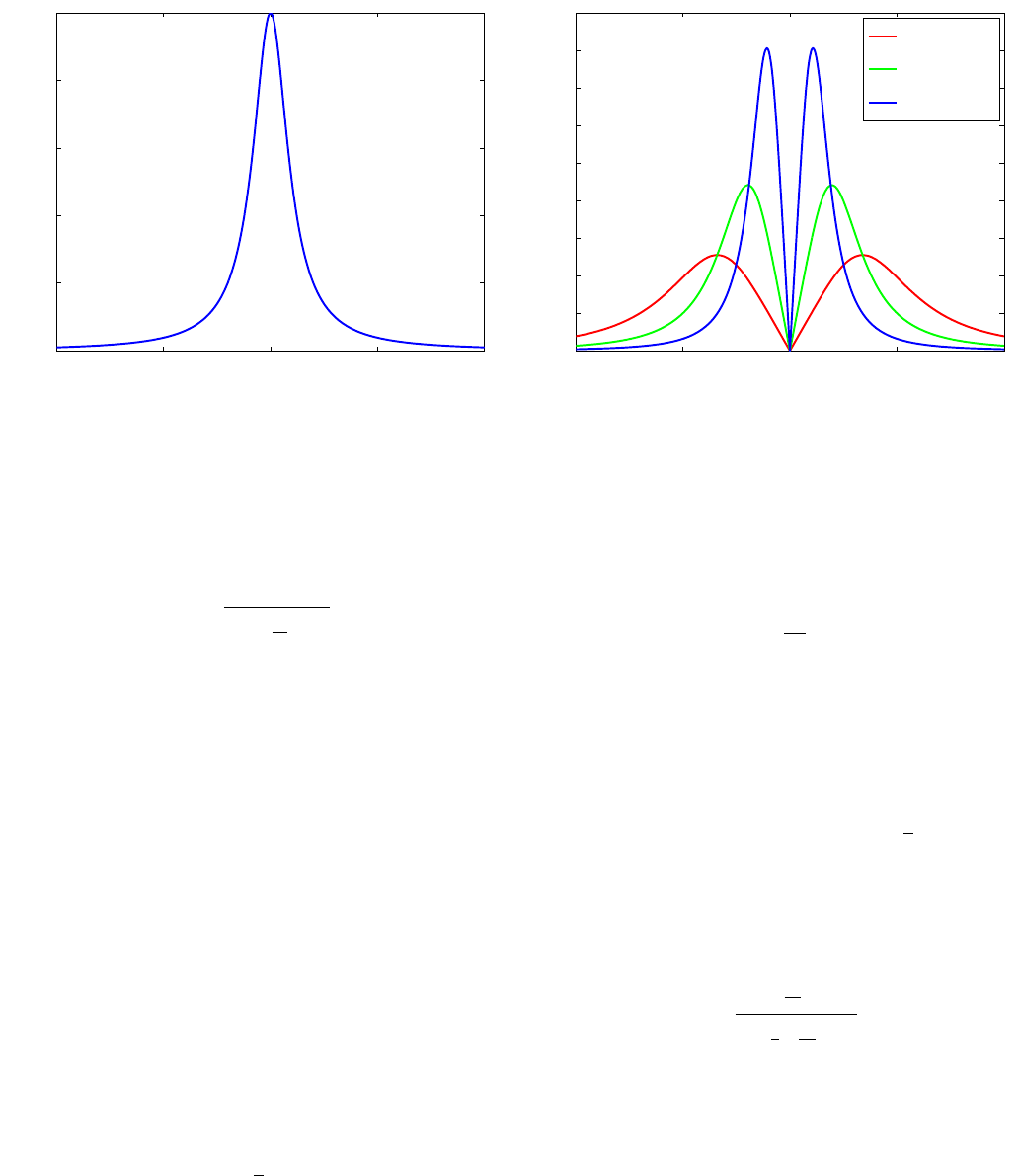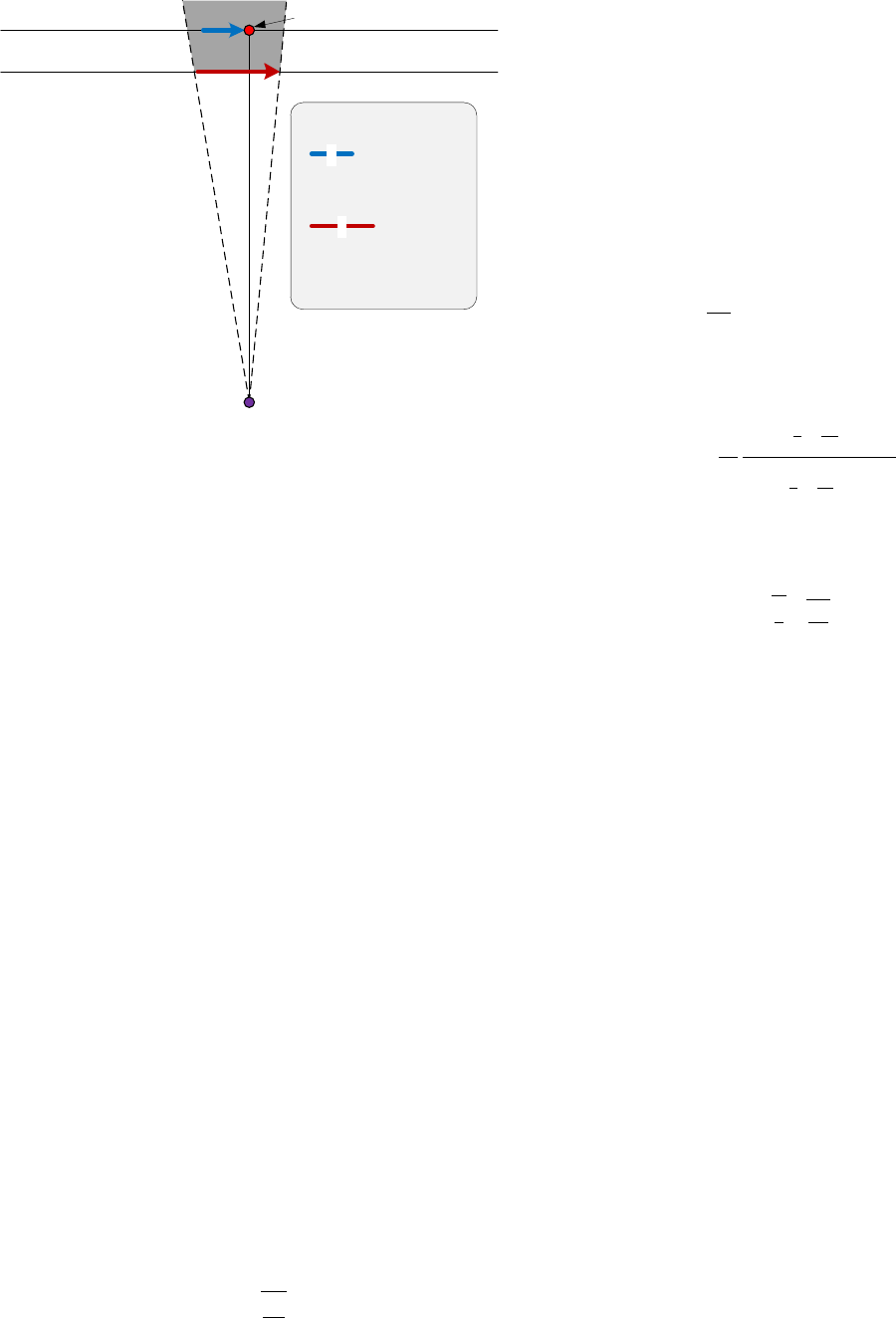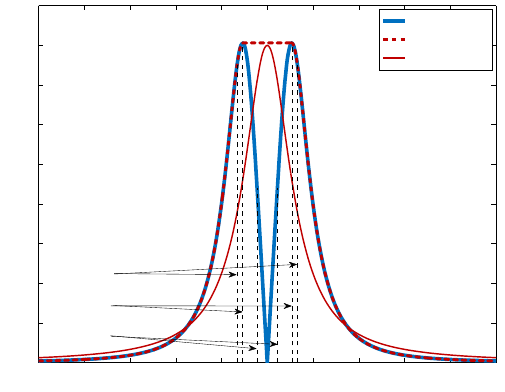### TL;DR In 2012, Dmitri Krioukov, a physicist at the Universit...
Prof. Dmitri Krioukov graduated with a Diploma in Physics from Sain...
[Here's an applet to illustrate dynamically the two situations that...
Krioukov told PhysicsCentral that the case and his argument were re...
The conclusions make sense, but only if you accept that the length ...The Proof of Innocence
Dmitri Krioukov
1
1
Cooperative Association for Internet Data Analysis (CAIDA),
University of California, San Diego (UCSD), La Jolla, CA 92093, USA
We show that if a car stops at a stop sign, an observer, e.g., a police oﬃcer, located at a certain
distance perpendicular to the car trajectory, must have an illusion that the car does not stop, if the
following three conditions are satisﬁed: (1) the observer measures not the linear but angular speed
of the car; (2) the car decelerates and subsequently accelerates relatively fast; and (3) there is a
short-time obstruction of the observer’s view of the car by an external object, e.g., another car, at
the moment when both cars are near the stop sign.
I. INTRODUCTION
It is widely known that an observer measuring the
speed of an object passing by, measures not its actual
linear velocity by the angular one. For example, if we
stay not far away from a railroad, watching a train ap-
proaching us from far away at a constant speed, we ﬁrst
perceive the train not moving at all, when it is really far,
but when the train comes closer, it appears to us mov-
ing faster and faster, and when it actually passes us, its
visual speed is maximized.
This observation is the ﬁrst building block of our proof
of innocence. To make this proof rigorous, we ﬁrst con-
sider the relationship between the linear and angular
speeds of an object in the toy example where the ob-
ject moves at a constant linear speed. We then proceed
to analyzing a picture reﬂecting what really happened
in the considered case, that is, the case where the linear
speed of an object is not constant, but what is constant
instead is the deceleration and subsequent acceleration of
the object coming to a complete stop at a point located
closest to the observer on the object’s linear trajectory.
Finally, in the last section, we consider what happens
if at that critical moment the observer’s view is brieﬂy
obstructed by another external object.
II. CONSTANT LINEAR SPEED
Consider Fig. 1 schematically showing the geometry of
the considered case, and assume for a moment that C’s
linear velocity is constant in time t,
v(t) v
0
. (1)
Without loss of generality we can choose time units t such
that t = 0 corresponds to the moment when C is at S.
Then distance x is simply
x(t) = v
0
t. (2)
Observer O visually measures not the linear speed of C
but its angular speed given by the ﬁrst derivative of an-
gle α with respect to time t,
ω(t) =
dt
. (3)
O (police officer)
S (stop sign)C (car)
r
0
L (lane)
α
v
x
FIG. 1: The diagram showing schematically the geometry of
the considered case. Car C moves along line L. Its current
linear speed is v, and the current distance from stop sign S
is x, |CS| = x. Another road connects to L perpendicularly
at S. Police oﬃcer O is located on that road at distance r
0
from the intersection, |OS| = r
0
. The angle between OC and
OS is α.
To express α(t) in terms of r
0
and x(t) we observe from
triangle OCS that
tan α(t) =
x(t)
r
0
, (4)
α(t) = arctan
x(t)
r
0
. (5)
Substituting the last expression into Eq. (3) and using
the standard diﬀerentiation rules there, i.e., speciﬁcally
the fact that
d
dt
arctan f(t) =
1
1 + f
2
df
dt
, (6)
where f(t) is any function of t, but it is f(t) = v
0
t/r
0
here, we ﬁnd that the angular speed of C that O observes
arXiv:1204.0162v1 [physics.pop-ph] 1 Apr 20122
−10 −5 0 5 1
0
0
0.2
0.4
0.6
0.8
1
t (seconds)
FIG. 2: The angular velocity ω of C observed by O as a
function of time t if C moves at constant linear speed v
0
. The
data is shown for v
0
= 10 m/s = 22.36 mph and r
0
= 10 m =
32.81 ft.
as a function of time t is
ω(t) =
v
0
/r
0
1 +
v
0
r
0
2
t
2
. (7)
This function is shown in Fig. 2. It conﬁrms and quan-
tiﬁes the observation discussed in the previous section,
that at O, the visual angular speed of C moving at a
constant linear speed is not constant. It is the higher,
the closer C to O, and it goes over a sharp maximum at
t = 0 when C is at the closest point S to O on its linear
trajectory L.
III. CONSTANT LINEAR DECELERATION
AND ACCELERATION
In this section we consider the situation closely mim-
icking what actually happened in the considered case.
Speciﬁcally, C, instead of moving at constant linear
speed v
0
, ﬁrst decelerates at constant deceleration a
0
,
then comes to a complete stop at S, and ﬁnally acceler-
ates with the same constant acceleration a
0
.
In this case, distance x(t) is no longer given by Eq. (2).
x(t) =
1
2
a
0
t
2
. (8)
If this expression does not look familiar, it can be easily
derived. Indeed, with constant deceleration/acceleration,
the velocity is
v(t) = a
0
t, (9)
−10 −5 0 5 1
0
0
0.1
0.2
0.3
0.4
0.5
0.6
0.7
0.8
0.9
t (seconds)
a
0
= 1 m/s
2
a
0
= 3 m/s
2
a
0
= 10 m/s
2
FIG. 3: The angular velocity ω of C observed by O as a func-
tion of time t if C moves with constant linear deceleration a
0
,
comes to a complete stop at S at time t = 0, and then moves
with the same constant linear acceleration a
0
. The data are
shown for r
0
= 10 m.
but by the deﬁnition of velocity,
v(t) =
dx
dt
, (10)
so that
dx = v(t) dt. (11)
Integrating this equation we obtain
x(t) =
Z
x
0
dx =
Z
t
0
v(t) dt = a
0
Z
t
0
t dt =
1
2
a
0
t
2
. (12)
Substituting the last expression into Eq. (5) and then dif-
ferentiating according to Eq. (3) using the rule in Eq. (6)
with f (t) = a
0
t
2
/(2r
0
), we obtain the angular velocity of
C that O observes
ω(t) =
a
0
r
0
t
1 +
1
4
a
0
r
0
2
t
4
. (13)
This function is shown in Fig. 3 for diﬀerent values
of a
0
. In contrast to Fig. 2, we observe that the angular
velocity of C drops to zero at t = 0, which is expected
because C comes to a complete stop at S at this time.
However, we also observe that the higher the decelera-
tion/acceleration a
0
, the more similar the curves in Fig. 3
become to the curve in Fig. 2. In fact, the blue curve in
Fig. 3 is quite similar to the one in Fig. 2, except the nar-
row region between the two peaks in Fig. 3, where the
angular velocity quickly drops down to zero, and then
quickly rises up again to the second maximum.3
O (police officer)
S (stop sign)C
1
(car #1)
r
0
L
1
(lane #1)
L
2
(lane #2)
C
2
(car #2)
l
1
C
1
= Toyota Yaris
l
1
= 150 in
l
2
C
2
Subaru Outback
l
2
= 189 in
l
2
l
1
= 39 in 1 m
FIG. 4: The diagram showing schematically the brief obstruc-
tion of view that happened in the considered case. The O’s
observations of car C
1
moving in lane L
1
are brieﬂy obstructed
by another car C
2
moving in lane L
2
when both cars are near
stop sign S. The region shaded by the grey color is the area
of poor visibility for O.
IV. BRIEF OBSTRUCTION OF VIEW AROUND
t = 0.
Finally, we consider what happens if the O’s observa-
tions are brieﬂy obstructed by an external object, i.e.,
another car, see Fig. 4 for the diagram depicting the
considered situation. The author/defendant (D.K.) was
driving Toyota Yaris (car C
1
in the diagram), which is
one of the shortest cars avaialable on the market. Its
lengths is l
1
= 150 in. (Perhaps only the Smart Cars are
shorter?) The exact model of the other car (C
2
) is un-
known, but it was similar in length to Subaru Outback,
whose exact length is l
2
= 189 in.
To estimate times t
p
and t
f
at which the partial and,
respectively, full obstructions of view of C
1
by C
2
be-
gan and ended, we must use Eq. (8) substituting there
x
p
= l
2
+ l
1
= 8.16 m, and x
f
= l
2
l
1
= 0.99 m, re-
spectively. To use Eq. (8) we have to know C
1
’s decelera-
tion/acceleration a
0
. Unfortunately, it is diﬃcult to mea-
sure deceleration or acceleration without special tools,
but we can roughly estimate it as follows. D.K. was badly
sick with cold on that day. In fact, he was sneezing while
approaching the stop sign. As a result he involuntary
pushed the brakes very hard. Therefore we can assume
that the deceleration was close to maximum possible for
a car, which is of the order of 10 m/s
2
= 22.36 mph/s.
We will thus use a
0
= 10 m/s
2
. Substituting these values
of a
0
, x
p
, and x
f
into Eq. (8) inverted for t,
t =
r
2x
a
0
, (14)
we obtain
t
p
= 1.31 s, (15)
t
f
= 0.45 s. (16)
The full durations of the partial and full obstructions are
then just double these times.
Next, we are interested in time t
0
at which the angular
speed of C
1
observed by O without any obstructions goes
over its maxima, as in Fig. 3. The easiest way to ﬁnd t
0
is to recall that the value of the ﬁrst derivative of the
angular speed at t
0
is zero,
dt
= ˙ω(t
0
) = 0. (17)
To ﬁnd ˙ω(t) we just diﬀerentiate Eq. (13) using the stan-
dard diﬀerentiation rules, which yield
˙ω(t) = 4
a
0
r
0
1
3
4
a
0
r
0
2
t
4
1 +
1
4
a
0
r
0
2
t
4
2
. (18)
This function is zero only when the numerator is zero, so
that the root of Eq. (17) is
t
0
=
4
r
4
3
r
r
0
a
0
. (19)
Substituting the values of a
0
= 10 m/s
2
and r
0
= 10 m
in this expression, we obtain
t
0
= 1.07 s. (20)
We thus conclude that time t
0
lies between t
f
and t
p
,
t
f
< t
0
< t
p
, (21)
and that diﬀerences between all these times is actually
quite small, compare Eqs. (15,16,20).
These ﬁndings mean that the angular speed of C
1
as
observed by O went over its maxima when the O’s view
of C
1
was partially obstructed by C
2
, and very close in
time to the full obstruction. In lack of complete informa-
tion, O interpolated the available data, i.e., the data for
times t > t
0
t
f
t
p
, using the simplest and physiologi-
cally explainable linear interpolation, i.e., by connecting
the boundaries of available data by a linear function. The
result of this interpolation is shown by the dashed curve
in Fig. 5. It is remarkably similar to the curve show-
ing the angular speed of a hypothetical object moving at
constant speed v
0
= 8 m/s 18 mph.
V. CONCLUSION
In summary, police oﬃcer O made a mistake, confusing
the real spacetime trajectory of car C
1
—which moved
at approximately constant linear deceleration, came to a4
−10 −8 −6 −4 −2 0 2 4 6 8 10
0
0.1
0.2
0.3
0.4
0.5
0.6
0.7
0.8
0.9
t (seconds)
C
1
’s speed
O’s perception
v
0
= 8 m/s
t
f
t
t
p
FIG. 5: The real angular speed of C
1
is shown by the blue solid
curve. The O’s interpolation is the dashed red curve. This
curve is remarkably similar to the red solid curve, showing
the angular speed of a hypothetical object moving at constant
linear speed v
0
= 8 m/s = 17.90 mph.
complete stop at the stop sign, and then started moving
again with the same acceleration, the blue solid line in
Fig. 5—for a trajectory of a hypothetical object moving
at approximately constant linear speed without stopping
at the stop sign, the red solid line in the same ﬁgure.
However, this mistake is fully justiﬁed, and it was made
possible by a combination of the following three factors:
1. O was not measuring the linear speed of C
1
by any
special devices; instead, he was estimating the vi-
sual angular speed of C
1
;
2. the linear deceleration and acceleration of C
1
were
relatively high; and
3. the O’s view of C
1
was brieﬂy obstructed by an-
other car C
2
around time t = 0.
As a result of this unfortunate coincidence, the O’s per-
ception of reality did not properly reﬂect reality.
### TL;DR In 2012, Dmitri Krioukov, a physicist at the University of California, San Diego, faced a $\$400$fine for allegedly running a stop sign. Instead of accepting the fine, Krioukov crafted an academic paper titled "The Proof of Innocence" to argue his case in court. Surprisingly, the judge was convinced and acquitted him. Krioukov shared his paper online with a subtitle, "A way to fight your traffic tickets," humorously noting that the paper earned a special prize of$400, which he didn't have to pay to California. The paper discusses a scenario in which if a car stops at a stop sign, an observer (e.g., a police officer) located at a specific distance perpendicular to the car's trajectory might perceive the car as not stopping under specific conditions. These conditions are: 1 - The observer measures not the linear but angular speed of the car. 2 - The car decelerates and subsequently accelerates relatively quickly. 3 - There is a short-time obstruction of the observer's view of the car by an external object (e.g., another car) at the moment when both cars are near the stop sign. Disclaimer: the paper was submitted on April 1st, 2012, so there's a non-zero probability this could a be joke by Dmitri :) Either way, it's a good paper. Prof. Dmitri Krioukov graduated with a Diploma in Physics from Saint Petersburg State University in 1993, and earned his Ph.D. in Physics from Old Dominion University in 1998. He transitioned to the industry and later returned to academia, joining CAIDA at UCSD as a Senior Research Scientist. Since 2014, he's been an Associate Professor at Northeastern University, engaging in complex network theory research as the Director of the DK-Lab at the Network Science Institute. ![](https://coe.northeastern.edu/wp-content/uploads/profiles/affiliated/krioukov-d.jpg) The conclusions make sense, but only if you accept that the length of the car that obstructed the view of the police officer was similar to that of a Subaru Outback :) ![](https://cdn11.bigcommerce.com/s-pusehjx/images/stencil/1280x1280/products/6626/21101/ironman-Outback-woods-profile_1500x1000__93093.1683670385.jpg?c=2) [Here's an applet to illustrate dynamically the two situations that the author describes](https://www.geogebra.org/m/uh8kqbf4). You can alter the acceleration of the car and the distance away the observer is to see (from the arrows) how the angular speed changes. Krioukov told PhysicsCentral that the case and his argument were real, but that he left a flaw in his work for others to find. According to the manufacturer’s data, Toyota Yaris accelerates from 0-100 km/h in 4.8 seconds = 5.8 $m^2s^{-1}$, making it hard to believe in the 10 $m^2s^{-1}$ suggested by the author.